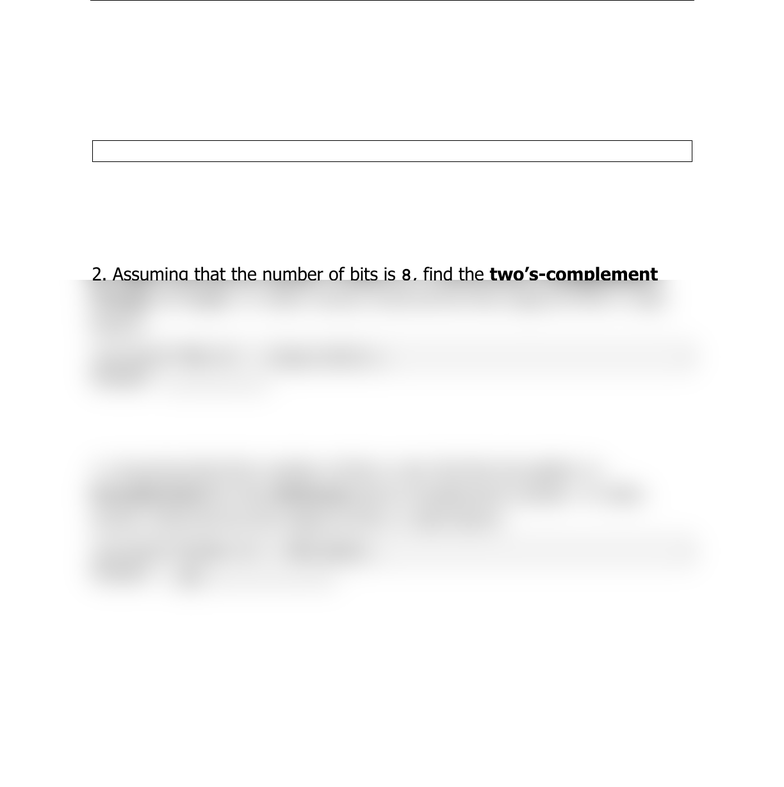Textbook Notes (280,000)
US (110,000)
LSU (20,000)
CSC (200)
All (7)
Chapter

# Quiz1

Department
Computer Science
Course Code
CSC 3501
Professor
All

This preview shows half of the first page. to view the full 1 pages of the document.CSC 3501 Quiz 1 (9/7/2017) A
Last name:
First name:
Some numbers you may want to use: 25=32, 26=64, 27=128, 28=256
1. Assuming that the number of bits is 8, find the unsinged integer for
0x82. In other words, what will be the output of the C code below?
printf("%u\n", (unsigned char)0x82);
2. Assuming that the number of bits is 8, find the two’s-complement
integer for 0x81. In other words, what will be the output of the C code
below?
printf("%d\n", (char)0x81);
3. Assuming that the number of bits is 32, find the bit pattern in
hexadecimal for the minimum two’s-complement number. In other
words, what will be the output of the C code below?
printf("0x%x\n", INT_MIN);